Function Repository Resource:

# SigmaConfidenceLevel

Calculate the confidence level corresponding to width around the mean of a normal distribution

Contributed by: Julien Kluge
 ResourceFunction["SigmaConfidenceLevel"][σ] calculates the fraction of the normal distribution that falls within σ standard deviations of the mean.

## Details and Options

ResourceFunction["SigmaConfidenceLevel"] evaluates to exact outputs for exact inputs and certain values.
The associated one-sided p-value can be calculated by using p=(1-ResourceFunction["SigmaConfidenceLevel"][σ])/2.

## Examples

### Basic Examples (2)

Calculate the confidence level at one standard deviation:

 In:=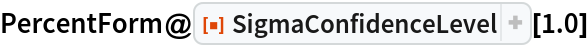Out=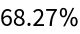Calculate the p-value associated with 3σ:

 In:=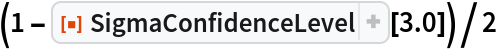Out=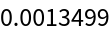### Scope (5)

SigmaConfidenceLevel returns exact outputs for exact inputs:

 In:=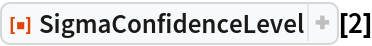Out=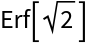In:=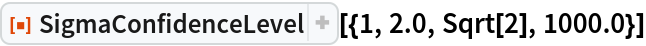Out=In physics, a discovery typically has to pass a five-σ of certainty test. Calculate the probability that a five-σ result is not a statistical fluctuation:

 In:=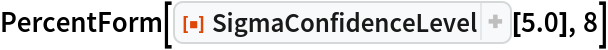Out=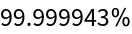A p<0.05 test corresponds to a maximum displacement from mean of about 1.64 standard deviations:

 In:=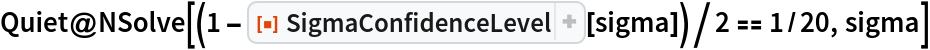Out=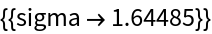SigmaConfidenceLevel can be defined as the integral between -σ and σ of the NormalDistribution with mean 0 and standard deviation 1:

 In:=Out=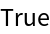### Applications (1)

Fit the mean prediction bands of a linear model fit with a confidence level of 1σ, 2σ and 3σ:

 In:=Out=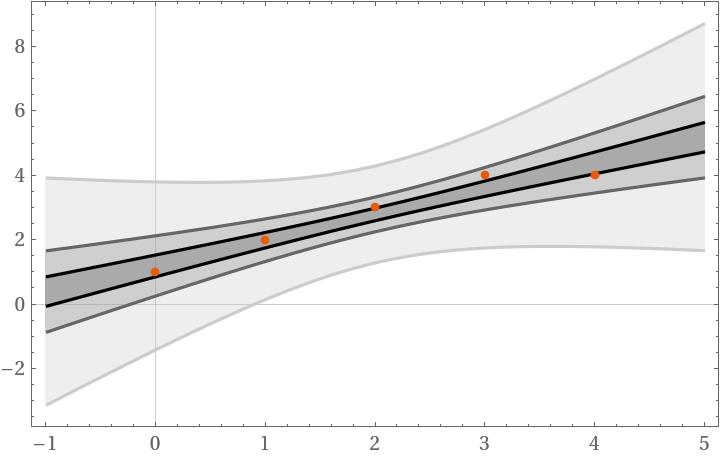### Properties and Relations (1)

SigmaConfidenceLevel handles infinities:

 In:=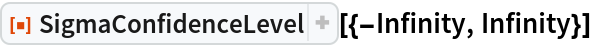Out=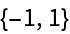### Neat Examples (1)

The 5σ classification limit in physics for discoveries has a false-positive probability of approximately:

 In:=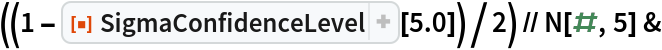Out=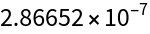Julien Kluge

## Version History

• 1.0.0 – 23 April 2020

## Author Notes

Julien Kluge Quantum Optical Metrology; Joint Lab Integrated Quantum Sensors Department of Physics Humboldt-Universität zu Berlin (julien@physik.hu-berlin.de)